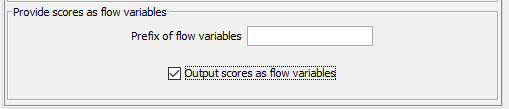# Polynomial Regression and Parameter Optimization

Hi all,

I’d like to use the X-Partitioner noce together with a Polynomial Regression Learner and the Parameter Optimization Loop.
The workflow without the loop is working fine. Now, I want to connect the Parameter Optimization Loop Start and the X-Partitioner.

Usage of the flow variable:

The Parameter Optimization Loop Start should be connectes to the Numeric Scorer (after the X-Aggregator).

Why is’nt it possible to minimize the root squared error of the statistics node?

Many thanks

It is possible. You just need to configure the Numeric Scorer node to output the scores as flow variables.2 Likes

ın my opinioon you should try to table row to verable node

for example

Thank you @elsamuel and @BerkayAkar.
Why should I use either the flow variable output or the table row to veriable node?

RegardsThe Parameter Optimization Loop End node requires a variable.

Therefore, if you want to minimize or maximize the output(s) from the Numeric Sorter node, then this must be converted to a variable.

Why try to do this with a separate node when the Numeric Scorer node itself can be set to output all the variables?

1 Like

so fi you use the table row verable you can looking best parameter for all optimized parameters

Many thanks!
For me the “easy” solution using the flow variable putput worked absolutely fineThank you both

This topic was automatically closed 7 days after the last reply. New replies are no longer allowed.